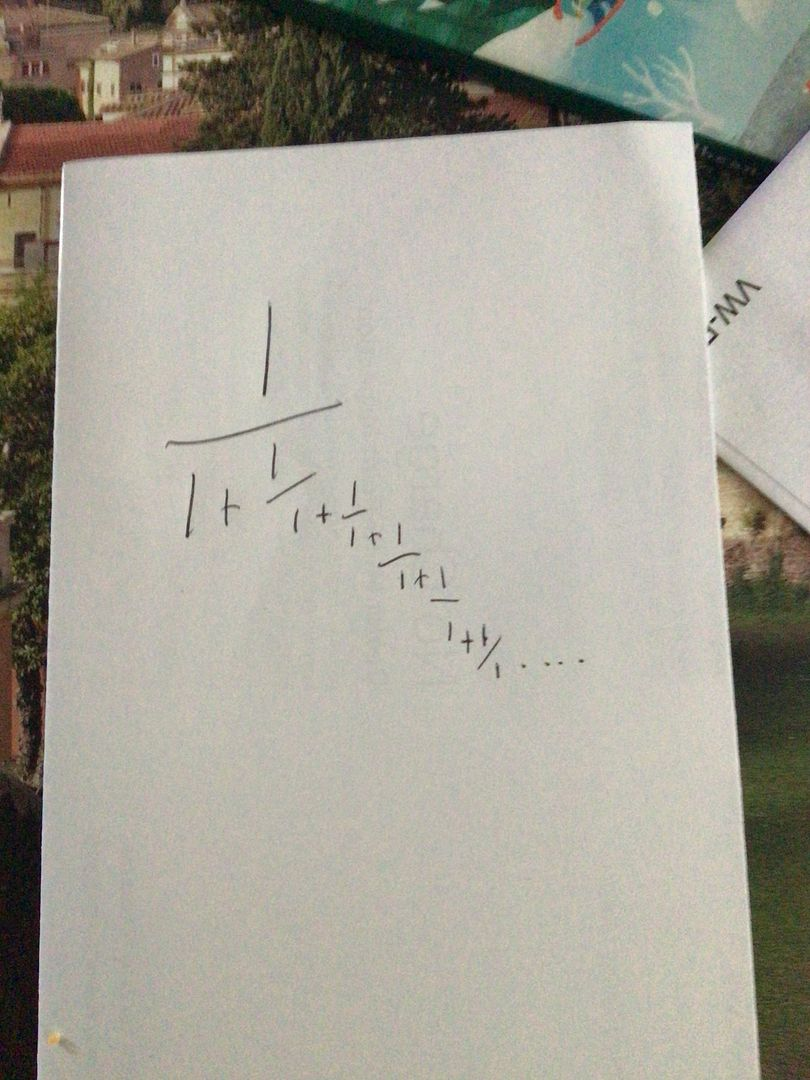MoscaJoined: Dec 14, 2009
• Posts: 3909
November 17th, 2021 at 7:59:28 AM permalink
I’m interested in the answer, but I’m more interested in the method for how to approach this. In the middle of a dream last night I thought of this number:And in the dream I was trying to figure out what number it was. I knew (in the dream) that it approached a limit, but since I was dreaming it just turned into one of those circular dreams where I kept getting dumped to the beginning.

It looks like it’s just a tick over 1/2, but how do I think about it to find out what it really is?
NO KILL I
ThatDonGuyJoined: Jun 22, 2011
• Posts: 5353
Thanks for this post from:November 17th, 2021 at 8:02:54 AM permalink
An easy one...

Let X = 1 / (1 + 1 / (1 + 1 / (1 + 1 / (....)

1 / X = 1 + 1 / (1 + 1 / (1 + 1 / (....)
1 / X - 1 = 1 / (1 + 1 / (1 + 1 / (....) = X
X - 1 / X + 1 = 0
X^2 + X - 1 = 0
X = -1/2 +/- sqrt(5)/2
but in this case, X > 0, so X = (sqrt(5) - 1) / 2
MoscaJoined: Dec 14, 2009
• Posts: 3909
November 17th, 2021 at 8:07:55 AM permalink
Quote: ThatDonGuy

An easy one...

Let X = 1 / (1 + 1 / (1 + 1 / (1 + 1 / (....)

1 / X = 1 + 1 / (1 + 1 / (1 + 1 / (....)
1 / X - 1 = 1 / (1 + 1 / (1 + 1 / (....) = X
X - 1 / X + 1 = 0
X^2 + X - 1 = 0
X = -1/2 +/- sqrt(5)/2
but in this case, X > 0, so X = (sqrt(5) - 1) / 2